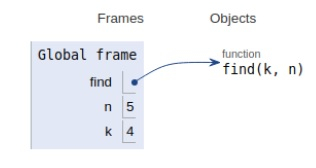# Python Program for nth multiple of a number in Fibonacci Series

Problem statement− We are given a number, we need to find the nth multiple of a number k in Fibonacci number.

The solution to the problem is discussed below−

## Example

Live Demo

# find function
def find(k, n):
f1 = 0
f2 = 1
i =2;
#fibonacci recursion
while i!=0:
f3 = f1 + f2;
f1 = f2;
f2 = f3;
if f2%k == 0:
return n*i
i+=1
return
# multiple of which number
n = 5;
# number
k = 4;
print("Position of n\'th multiple of k in""Fibonacci Series is: ", find(k,n));

## Output

Position of n'th multiple of k inFibonacci Series is:  30All the variables and functions are declared in the global scope as shown in the figure above.

## Conclusion

In this article, we have learned how we can find nth multiple of a number k in the Fibonacci series.